• 时间:
• 浏览:

# 1 无约束问题定义

min fo(x),其中fo(x)为二次可微凸函数。

# 2 迭代算法求解

x(k+1)=x(k)+s(k)+d(k)

d(k)为k时刻的搜索方向，s(k)为k时刻的搜索步长

1 确定下降方向d(k)
2 确定步长s(k)
3 修改x(k+1)=x(k)+s(k)+d(k)

# 3 搜索步长

## 1 精确搜索：

s(k) = argmin f(x(k) + td(k))其中t>=0

## 2 不精确搜索

### Amijo Rule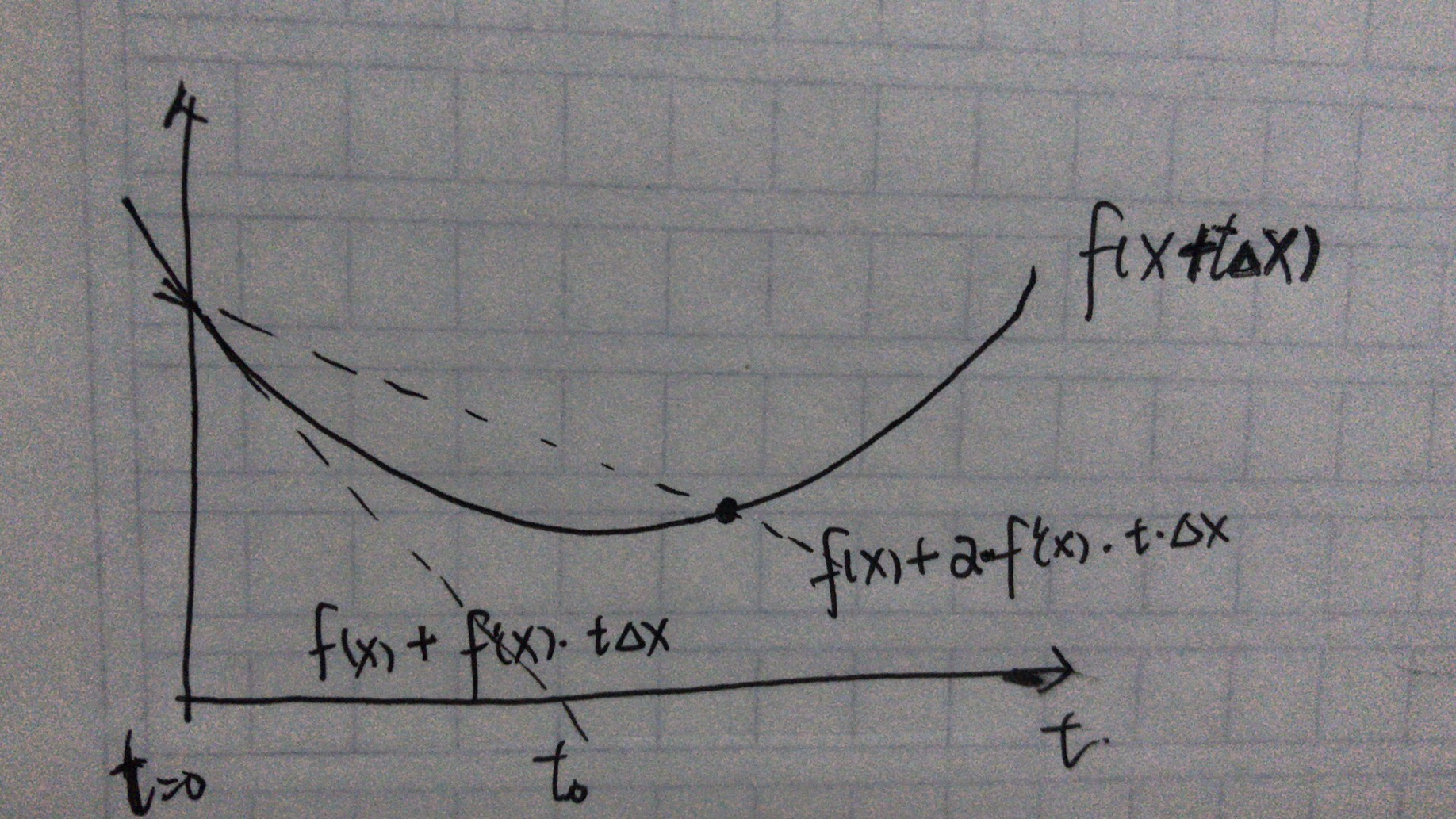# 4 离目标有多远

x’对应的值f(x’)与最优值p*有多远

f(y)=f(x)+f’(x)(y-x)+0.5 f’’(x)(y-x)2
==》f(y)>=f(x)+f’(x)(y-x)+m/2*||(y-x)||2

## 当f(x)的导数趋近于0时，f(x’)与最优值p*有多远

f’(x)+m(y-x)= 0,得到y再带入不等式，有
f(y)>=f(x)-1/(2m)*f’(x)2

## 当f(x)的导数趋近于0时，x’与最优解x*有多远

p* = f(x’) >= f(x)+f’(x)(x’-x)+m/2*||(x’-x)||2
>= f(x)+f’(x)||x’-x||+m/2*||(x’-x)||2

## 总结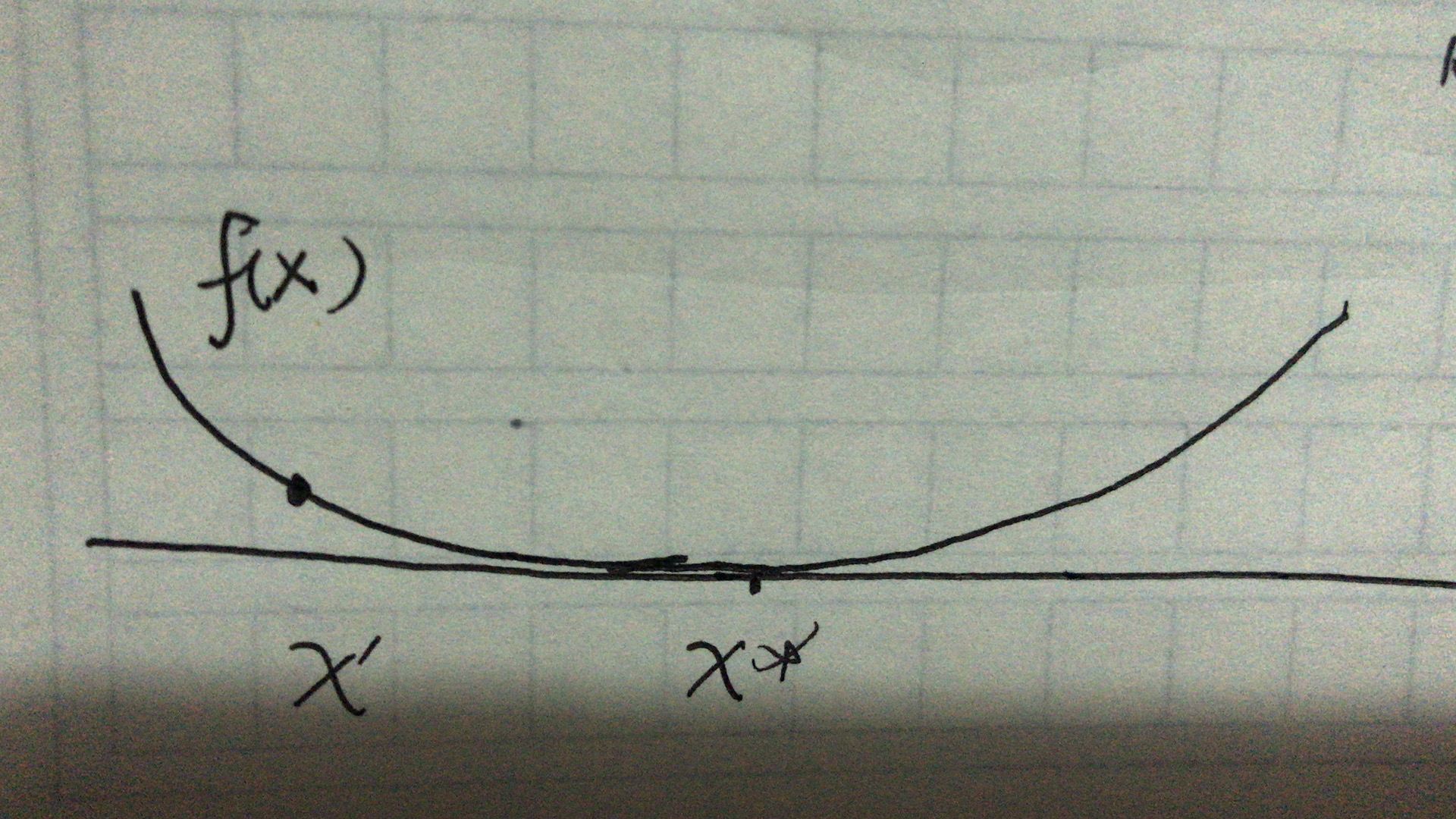# 5 搜索方向

## 2 最速下降法

f(x+v) = f(x)+f’(x)v,第二项为f在x处沿方向v的方向导数。定义一个规范化的最速下降方向
d = argmin{f’(x)v| v的范数为1}

## 3 梯度下降与最速下降法变种

### 次梯度下降法：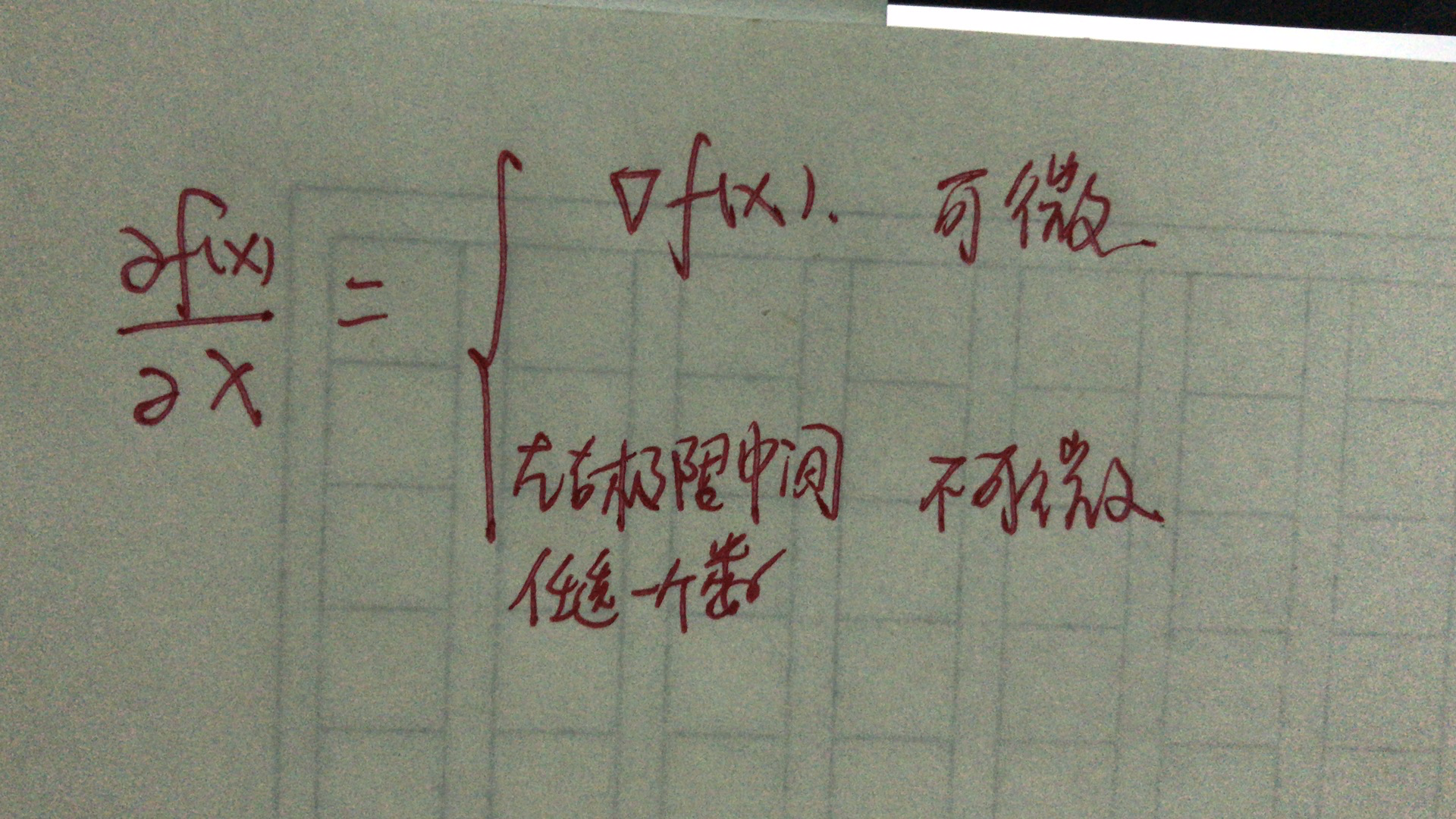## 4 牛顿法

f(x+v) = f(x)+f’(x)v+0.5f’’(x)v2

-[f’’(x)]-1f’(x)

## 5 拉格朗日法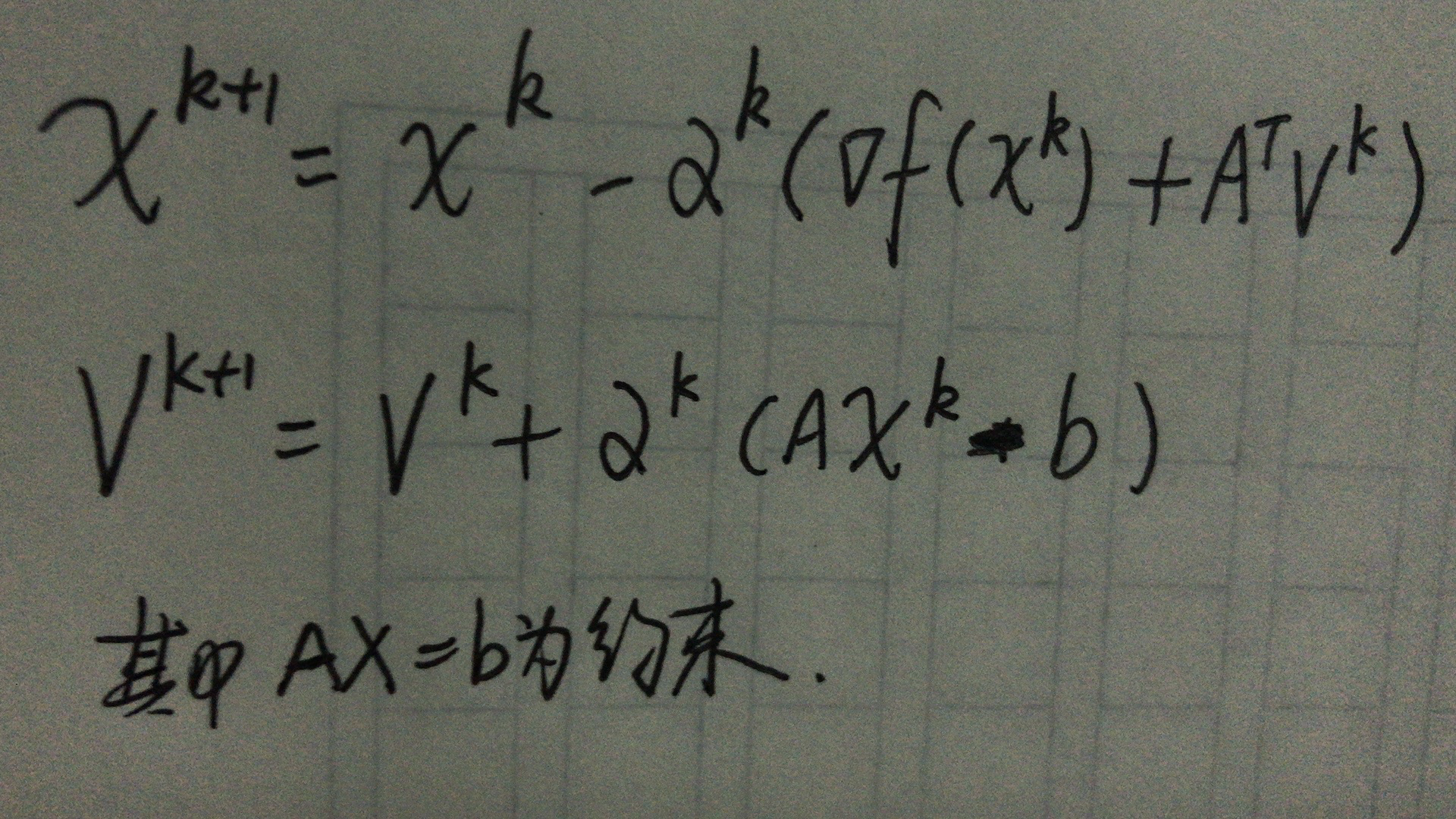## 6 增广的拉格朗日法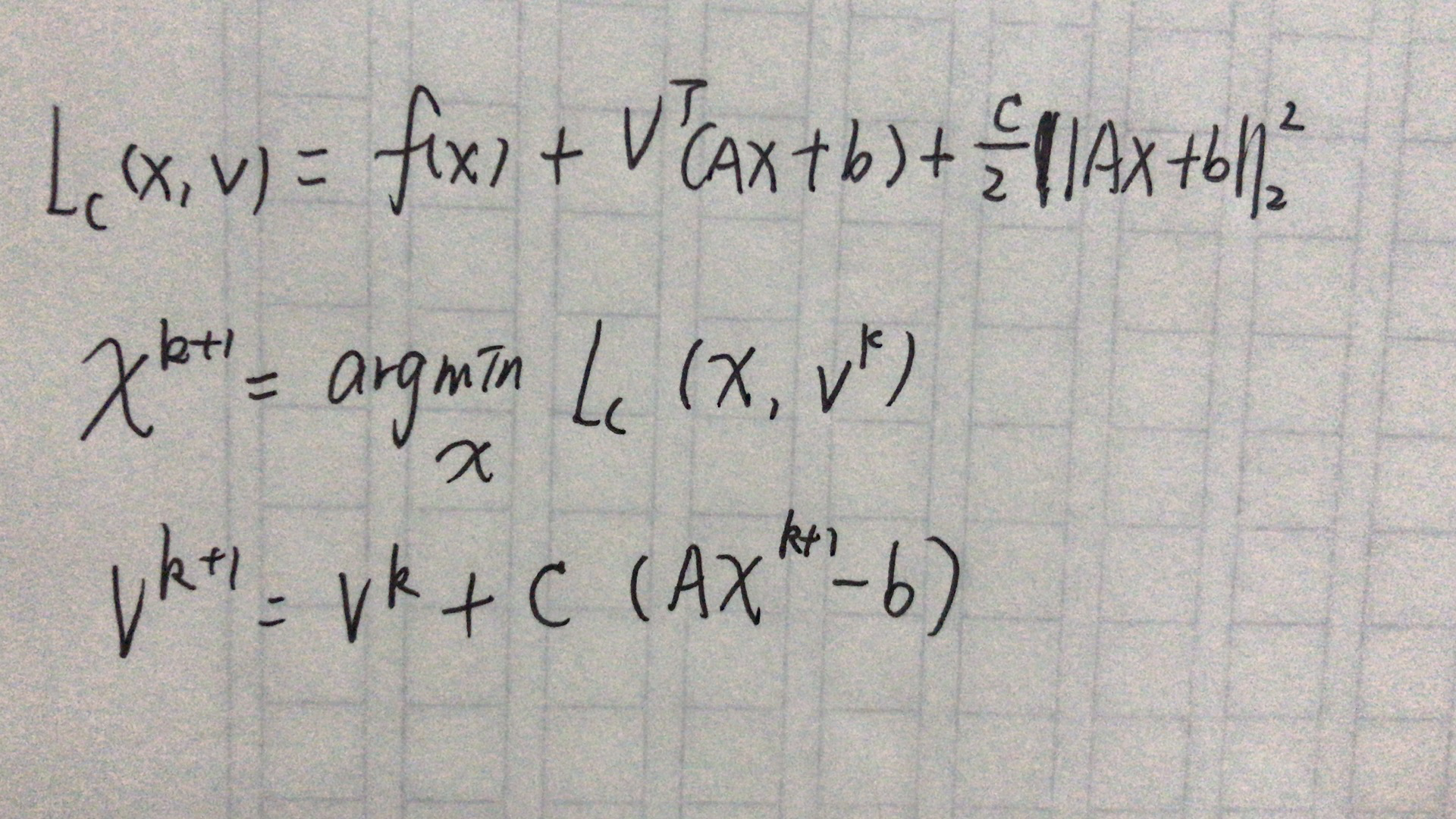、《凸优化》，Stephen Boyd等著，王书宁等译
https://blog.csdn.net/itplus/article/details/21896453
https://blog.csdn.net/itnerd/article/details/86012869